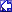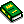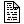AIX Documentation: Programmer's Reference:
Z

Current View: Programmer's Reference
Views: [ Books | Commands | Programmer's Reference | Tasks and Topics ]BackProgrammer's Reference
ZZAXPY Computes a constant times a vector plus a vector.ZCOPY Copies vector X to Y.ZDOTC Returns the complex dot product of two vectors, conjugating the first.ZDOTU Returns the complex dot product of two vectors.ZDROT Applies a plane rotation.ZDSCAL Scales a vector by a constant.ZGBMV Performs matrix-vector operations with general banded matrices.ZGEMM Performs matrix-matrix operations on general matrices.ZGEMV Performs matrix-vector operation with general matrices.ZGERC Performs the rank 1 operation.ZGERU Performs the rank 1 operation.ZHBMV Performs matrix-vector operations using a Hermitian band matrix.ZHEMM Performs matrix-matrix operations on Hermitian matrices.ZHEMV Performs matrix-vector operations using Hermitian matrices.ZHER Performs the Hermitian rank 1 operation.ZHER2 Performs the Hermitian rank 2 operation.ZHER2K Performs Hermitian rank 2k operations.ZHERK Performs Hermitian rank k operations.ZHPMV Performs matrix-vector operations using a packed Hermitian matrix.ZHPR Performs the Hermitian rank 1 operation.ZHPR2 Performs the Hermitian rank 2 operation.ZROTG Constructs Givens plane rotation.ZSCAL Scales a vector by a constant.ZSWAP Interchanges vectors X and Y.ZSYMM Performs matrix-matrix matrix operations on symmetric matrices.ZSYR2K Performs symmetric rank 2k operations.ZSYRK Perform symmetric rank k operations.ZTBMV Performs matrix-vector operations using a triangular band matrix.ZTBSV Solves system of equations.ZTPMV Performs matrix-vector operations on a packed triangular matrix.ZTPSV Solves systems of equations.ZTRMM Performs matrix-matrix operations on triangular matrices.ZTRMV Performs matrix-vector operations using a triangular matrix.ZTRSM Solves certain matrix equations.ZTRSV Solves system of equations.